International
Tables for
Crystallography
Volume B
Reciprocal space
Edited by U. Shmueli

International Tables for Crystallography (2010). Vol. B, ch. 2.5, pp. 303-304   | 1 | 2 |

## Section 2.5.2.6. Imaging with electrons

J. M. Cowleya

#### 2.5.2.6. Imaging with electrons

| top | pdf |

Electron optics. Electrons may be focused by use of axially symmetric magnetic fields produced by electromagnetic lenses. The focal length of such a lens used as a projector lens (focal points outside the lens field) is given by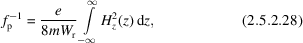where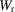is the relativistically corrected accelerating voltage andis the z component of the magnetic field. An expression in terms of experimental constants was given by Liebman (1955)as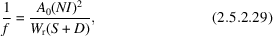whereis a constant, NI is the number of ampere turns of the lens winding, S is the length of the gap between the magnet pole pieces and D is the bore of the pole pieces.

Lenses of this type have irreducible aberrations, the most important of which for the paraxial conditions of electron microscopy is the third-order spherical aberration, coefficient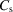, giving a variation of focal length of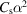for a beam at an angle α to the axis. Chromatic aberration, coefficient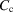, gives a spread of focal lengths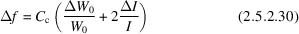for variationsandof the accelerating voltage and lens currents, respectively.

The objective lens of an electron microscope is the critical lens for the determination of image resolution and contrast. The action of this lens in a conventional transmission electron microscope (TEM) is described by use of the Abbe theory for coherent incident illumination transmitted through the object to produce a wavefunction(see Fig. 2.5.2.2).Figure 2.5.2.2 | top | pdf |Diagram representing the critical components of a conventional transmission electron microscope (TEM) and a scanning transmission electron microscope (STEM). For the TEM, electrons from a source A illuminate the specimen and the objective lens forms an image of the transmitted electrons on the image plane, B. For the STEM, a source at B is imaged by the objective lens to form a small probe on the specimen and some part of the transmitted beam is collected by a detector at A.

The amplitude distribution in the back focal plane of the objective lens is writtenwhereis the Fourier transform ofand T(u, v) is the transfer function of the lens, consisting of an aperture function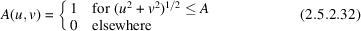and a phase function expwhere the phase perturbationdue to lens defocusand aberrations is usually approximated as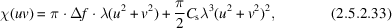and u, v are the reciprocal-space variables related to the scattering angles,byThe image amplitude distribution, referred to the object coordinates, is given by Fourier transform of (2.5.2.31)aswhere, given by Fourier transform of, is the spread function. The image intensity is thenIn practice the coherent imaging theory provides a good approximation but limitations of the coherence of the illumination have appreciable effects under high-resolution imaging conditions.

The variation of focal lengths according to (2.5.2.30)is described by a function. Illumination from a finite incoherent source gives a distribution of incident-beam angles. Then the image intensity is found by integrating incoherently overand: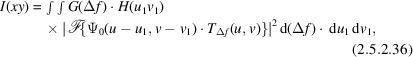wheredenotes the Fourier-transform operation.

In the scanning transmission electron microscope (STEM), the objective lens focuses a small bright source of electrons on the object and directly transmitted or scattered electrons are detected to form an image as the incident beam is scanned over the object (see Fig. 2.5.2.2). Ideally the image amplitude can be related to that of the conventional transmission electron microscope by use of the reciprocity relationship' which refers to point sources and detectors for scalar radiation in scalar fields with elastic scattering processes only. It may be stated: The amplitude at a point B due to a point source at A is identical to that which would be produced at A for the identical source placed at B'.

For an axial point source, the amplitude distribution produced by the objective lens on the specimen isIf this is translated by the scan to X, Y, the transmitted wave isThe amplitude on the plane of observation following the specimen is thenand the image signal produced by a detector having a sensitivity function H(u, v) is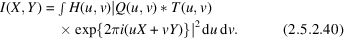If H(u, v) represents a small detector, approximated by a delta function, this becomeswhich is identical to the result (2.5.2.35)for a plane incident wave in the conventional transmission electron microscope.

### References

Liebman, G. (1955). A unified representation of magnetic electron lens properties. Proc. Phys. Soc. London Sect. B, 68, 737–745.